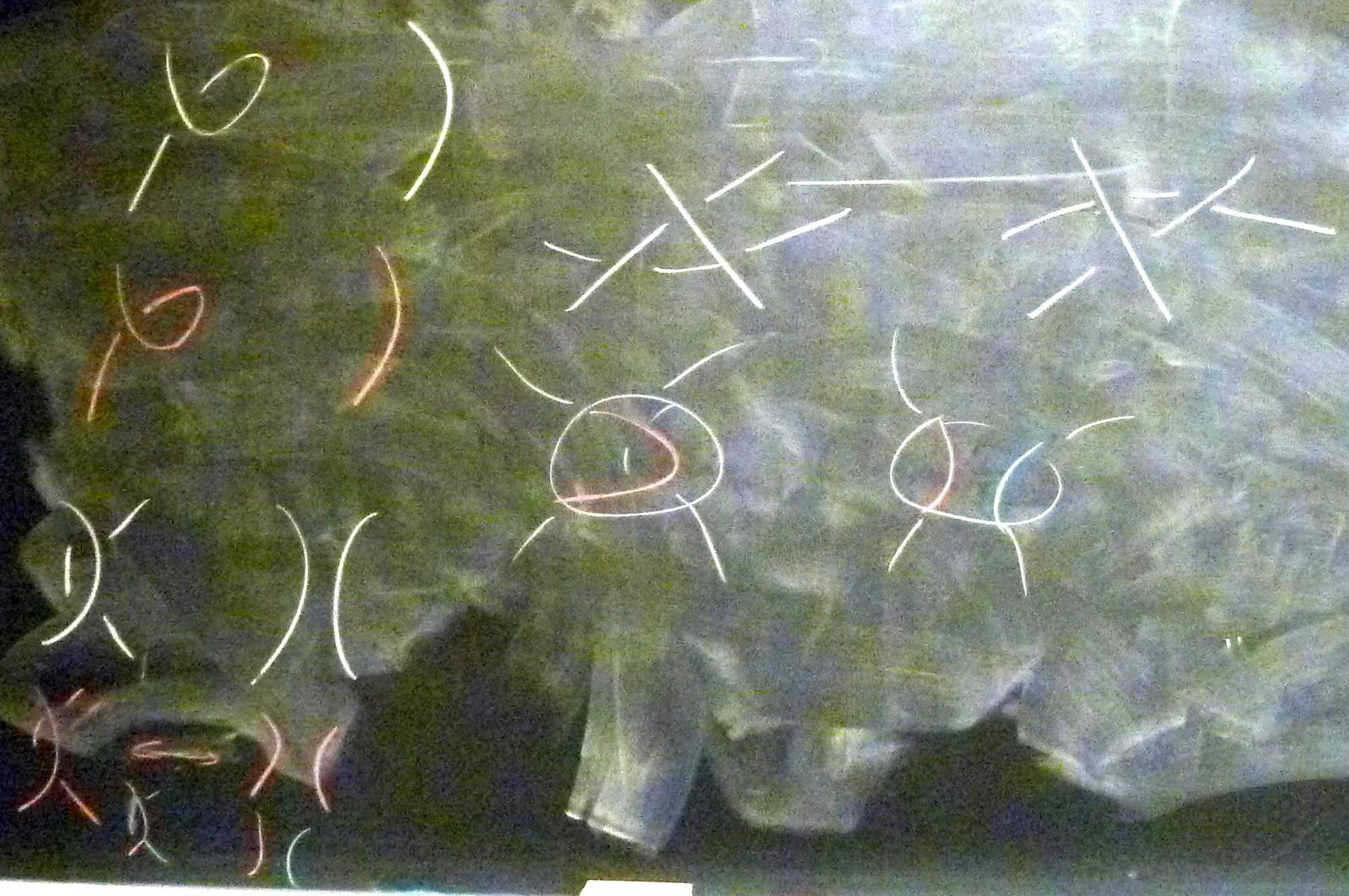© | Dror Bar-Natan: Classes: 2013-14: AKT: < >

# AKT-140106 Video

 width: 400 720 orig/AKT-140106.MOD For now, this video can only be viewed with web browsers that support

Notes on AKT-140106:    [edit, refresh]

Course introduction, knots and Reidemeister moves, knot colourings.

# Week of... Notes and Links
1 Jan 6 About This Class (PDF).
: Course introduction, knots and Reidemeister moves, knot colourings.
Tricolourability without Diagrams
: The Gauss linking number combinatorially and as an integral.
: The Schroedinger equation and path integrals.
Friday Introduction (the quantum pendulum)
2 Jan 13 Homework Assignment 1.
: The Kauffman bracket and the Jones polynomial.
: Self-linking using swaddling.
: Euler-Lagrange problems, Gaussian integration, volumes of spheres.
3 Jan 20 Homework Assignment 2.
: The definition of finite-type and some examples.
: The self-linking number and framings.
: Integrating a polynomial times a Gaussian.
Class Photo.
4 Jan 27 Homework Assignment 3.
: Chord diagrams and weight systems.
: Swaddling maps and framings, general configuration space integrals.
: Some analysis of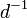$d^{-1}$.
5 Feb 3 Homework Assignment 4.
: 4T, the Fundamental Theorem and universal finite type invariants.
The Fulton-MacPherson Compactification (PDF).
: The Fulton-MacPherson Compactification, Part I.
: More on pushforwards,$d^{-1}$, and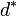$d^\ast$.
6 Feb 10 Homework Assignment 5.
: The bracket-rise theorem and the invariance principle.
: The Fulton-MacPherson Compactification, Part II.
: Gauge fixing, the beginning of Feynman diagrams.
R Feb 17 Reading Week.
7 Feb 24 : A review of Lie algebras.
: Graph cohomology and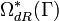$\Omega_{dR}^\ast(\Gamma)$.
: More on Feynman diagrams, beginning of gauge theory.
From Gaussian Integration to Feynman Diagrams (PDF).
8 Mar 3 Homework Assignment 6 (PDF)
: Lie algebraic weight systems.
: Graph cohomology and the construction of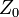$Z_0$.
Graph Cohomology and Configuration Space Integrals (PDF)
: Gauge invariance, Chern-Simons, holonomies.
Mar 9 is the last day to drop this class.
9 Mar 10 Homework Assignment 7 (PDF)
: The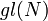$gl(N)$ weight system.
: The universal property, hidden faces.
: Insolubility of the quintic, naive expectations for CS perturbation theory.
10 Mar 17 Homework Assignment 8 (PDF)
: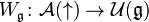$W_{\mathfrak g}\colon{\mathcal A}(\uparrow)\to{\mathcal U}({\mathfrak g})$ and PBW.
: The anomaly.
: Faddeev-Popov, part I.
Gaussian Integration, Determinants, Feynman Diagrams (PDF).
11 Mar 24 Homework Assignment 9 (PDF)
: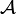${\mathcal A}$ is a bi-algebra.
: Understanding and fixing the anomaly.
Friday: class cancelled.
12 Mar 31 Monday, Wednesday: class cancelled.
: A Monday class: back to expansions.
E Apr 7 : A Friday class on what we mostly didn't have time to do.Add your name / see who's in!
Dror's Notebookrefresh
panel
Managed by dbnvp: Click "Comment on h:mm:ss" below the video to add a comment on a specific time.0:43:23  (Note by User:Cameron.martin):

Claim: The number of legal 3-colorings of a knot diagram is always a power of 3.

This is an expansion on the proof given by Przytycki (https://arxiv.org/abs/math/0608172).

We'll show that the set of legal 3-colorings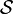$\mathcal{S}$ forms a subgroup of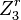$Z_3^r$, for some r, which suffices to prove the claim. First, label each of the segments of the given diagram 1 through n, and denote a 3-coloring of this diagram by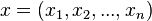$x = (x_1, x_2, ..., x_n)$, where each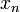$x_n$ is an element of the cyclic group of order 3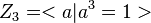$Z_3 = $ (each element representing a different colour). It is clear that$\mathcal{S}$ is a subset of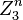$Z_3^n$. To show it is a subgroup, we'll take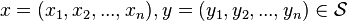$x = (x_1, x_2, ..., x_n), y = (y_1, y_2, ..., y_n) \in \mathcal{S}$, and show that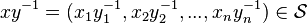$xy^{-1} = (x_1y_1^{-1}, x_2y_2^{-1}, ..., x_ny_n^{-1}) \in \mathcal{S}$. It suffices to restrict our attention to one crossing in the given diagram, so we can without loss of generality let n = 3.

First, we (sub)claim that a crossing (involving colours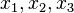$x_1, x_2, x_3$ is legal if and only if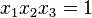$x_1x_2x_3 = 1$ in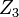$Z_3$. Indeed, if the crossing is legal, either it is the trivial crossing in which case their product is clearly 1, or each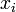$x_i$ is distinct, in which case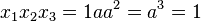$x_1x_2x_3 = 1aa^2 = a^3 = 1$. Conversely, suppose$x_1x_2x_3 = 1$, and suppose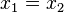$x_1 = x_2$. It suffices to show that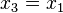$x_3 = x_1$. This follows by case checking: if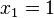$x_1 = 1$, then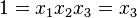$1 = x_1x_2x_3 = x_3$; if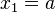$x_1 = a$, then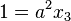$1=a^2x_3$, implying that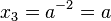$x_3 = a^{-2} = a$; and if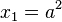$x_1 = a^2$, then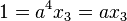$1 = a^4x_3 = ax_3$, implying that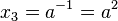$x_3 = a^{-1} = a^2$. Thus, the subclaim is proven.

As a result,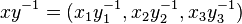$xy^{-1} = (x_1y_1^{-1}, x_2y_2^{-1}, x_3y_3^{-1})$ satisfies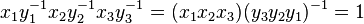$x_1y_1^{-1}x_2y_2^{-1}x_3y_3^{-1} = (x_1x_2x_3)(y_3y_2y_1)^{-1} = 1$ since both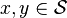$x, y \in \mathcal{S}$. This implies that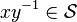$xy^{-1} \in \mathcal{S}$, and hence shows that$\mathcal{S}$ is a subgroup of$Z_3^n$ for n = the number of line segments in the diagram. By Lagrange's theorem, the number of legal 3-colorings (the order of$\mathcal{S}$) is a power of 3.

(Note by User:Leo algknt):

Using linear Algebra: Idea from class on Wednesday 23 May, 2018

Let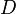$D$ be a knot diagram for the knot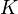$K$ with$n$ crossings. There are$n$ arcs. Let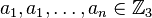$a_1, a_1, \ldots, a_n \in {\mathbb Z}_3$ represent the arcs. Now let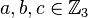$a,b,c \in {\mathbb Z}_3$. Define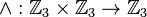$\wedge : {\mathbb Z}_3 \times {\mathbb Z}_3 \rightarrow {\mathbb Z}_3$ by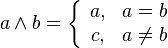$a\wedge b = \left\{ \begin{array}{cc} a, & a = b\\ c, & a\not= b \end{array} \right.$, so that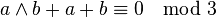$a\wedge b + a + b \equiv 0\mod 3$.

Then, with the above definition, we get a linear equation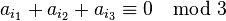$a_{i_1} + a_{i_2} + a_{i_3} \equiv 0\mod 3$ for each each of the$n$ crossings, where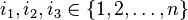$i_1, i_2, i_3 \in \{1, 2, \ldots, n\}$. Thus we get a system of$n$ linear equation, from which we get a matrix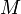$M$. The nullspace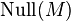$\mathrm{Null}(M)$ of$M$ is the solution to this system of equation and this is exactly the set of all 3-colourings of$D$. This is a vector space of size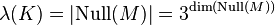$\lambda(K) =|\mathrm{Null}(M)| = 3^{\dim(\mathrm{Null}(M))}$0:46:29  (Note by User:Cameron.martin). The unknot $0_1$ and the figure-eight knot $4_1$ both have 3 legal 3-colorings, i.e. $\lambda(0_1) = \lambda(4_1) = 3$. 3-coloring fails to distinguish the unknot from the figure-eight. See http://katlas.math.toronto.edu/wiki/The_Rolfsen_Knot_Table for more information on specific knots.
0:51:18 [add] Reidemeister theorem and 3-colourings.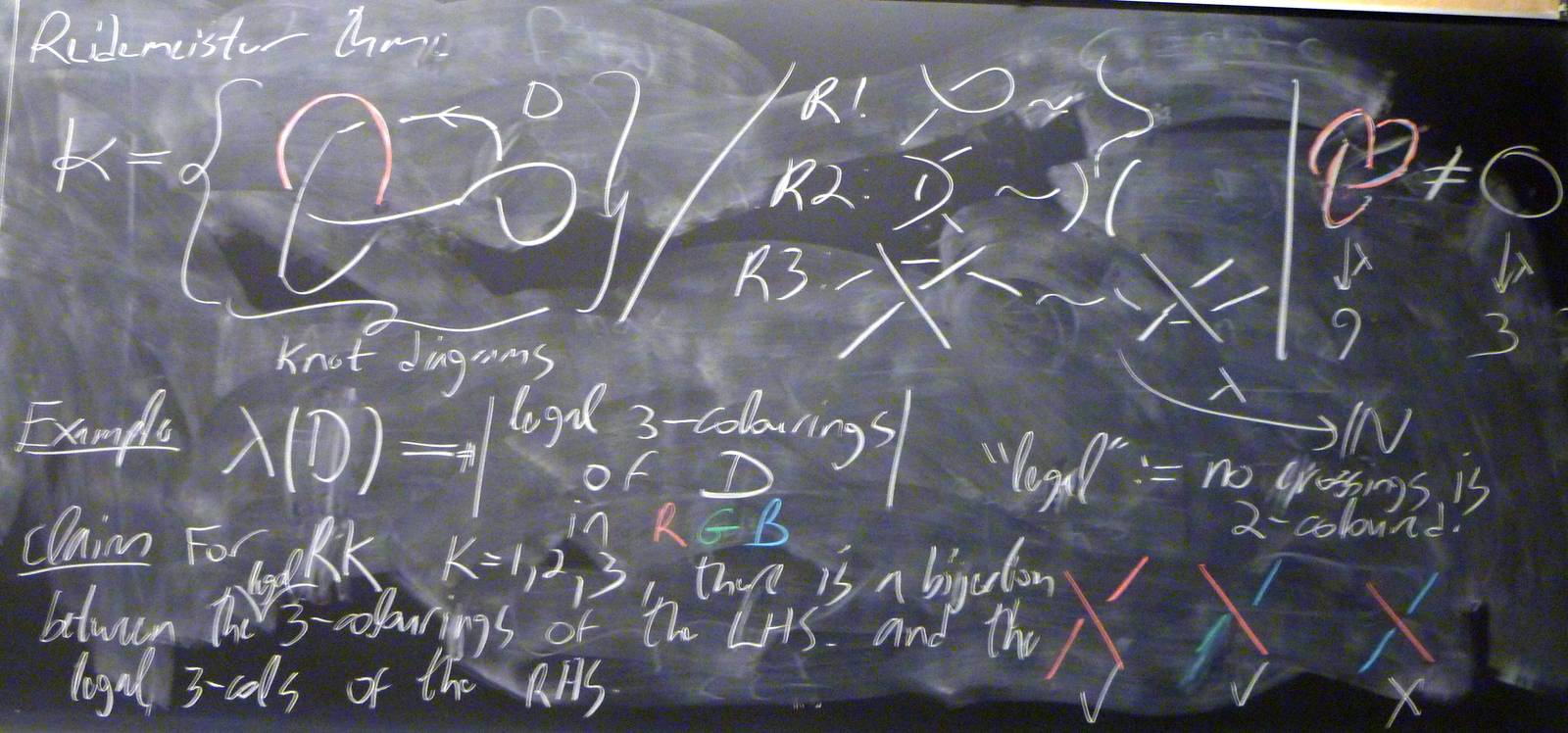0:55:56 [add] Invariance of 3-colourings under Reidemeister moves.